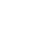# How to Find Third Friday of a Month in Python

Sharing is caring!

Last Updated on July 15, 2022 by Jay

In this tutorial, I’ll show you how to find the third Friday of a month in Python. Why the third Friday you ask? The third Friday is an important monthiversary for options traders – the monthly options expire on that day, so it’s kinda a big deal.

Let’s start by finding the third Friday for the current month (March), then generalize that.

## Finding the Third Friday of a Month

Python conveniently has a built-in library calendar, which is what we will use. Below shows:

• calendar.MONDAY = 0 (Python is 0 index)
• calendar.SUNDAY = 6 (this is the 7th day)
• calendar.FRIDAY = 4 (5th day)

However, we can still construct a Calendar object with SUNDAY being the first day of the week by using the firstweekday argument.

``````import calendar

print(calendar.SUNDAY)
6

print(calendar.MONDAY)
0

print(calendar.FRIDAY)
4

c = calendar.Calendar(firstweekday=calendar.SUNDAY)``````

Then we use the monthdatescalendar() method to get a list of the weeks in a given year and month. Each week is a list of 7 datetime.date objects representing the 7 days of a week.

Note the first day of the below calendar, “2022, 2, 27” matches the Windows calendar screenshot above.

``````c.monthdatescalendar(2022,3)

[[datetime.date(2022, 2, 27),datetime.date(2022, 2, 28),datetime.date(2022, 3, 1),
datetime.date(2022, 3, 2),datetime.date(2022, 3, 3),datetime.date(2022, 3, 4),
datetime.date(2022, 3, 5)],

[datetime.date(2022, 3, 6),datetime.date(2022, 3, 7),datetime.date(2022, 3, 8),
datetime.date(2022, 3, 9),datetime.date(2022, 3, 10),datetime.date(2022, 3, 11),
datetime.date(2022, 3, 12)],

[datetime.date(2022, 3, 13),datetime.date(2022, 3, 14),datetime.date(2022, 3, 15),
datetime.date(2022, 3, 16),datetime.date(2022, 3, 17),datetime.date(2022, 3, 18),
datetime.date(2022, 3, 19)],

[datetime.date(2022, 3, 20),
datetime.date(2022, 3, 21),datetime.date(2022, 3, 22),
datetime.date(2022, 3, 23),datetime.date(2022, 3, 24),datetime.date(2022, 3, 25),
datetime.date(2022, 3, 26)],

[datetime.date(2022, 3, 27),datetime.date(2022, 3, 28),datetime.date(2022, 3, 29),
datetime.date(2022, 3, 30),datetime.date(2022, 3, 31),datetime.date(2022, 4, 1),
datetime.date(2022, 4, 2)]]``````

Also conveniently, the week positions also match perfectly with a real calendar. The third Friday is in the 3rd list (index position of 2) and 6th item (index position of 5) because the weeks start from Sundays:

``````c.monthdatescalendar(2022,3)
datetime.date(2022, 3, 18)``````

## Special Case

The above logic works except for a special case – when the first day of a month is a Saturday. For example, Jan and October 2022 are examples of such a special case.

In this case, if we still set firstweekday =calendar.SUNDAY, it’s going to consider the week ending Oct 1 (a Saturday) as the first week. This is okay except that there’s NO Friday in that first week!

Therefore, the logic we looked at would return Oct 14 as the third Friday, despite it’s actually the 2nd Friday! Thanks to our reader Luca for pointing this out!

We can tweak the logic a little bit by setting fristweekday=calendar.SATURDAY to work around this special case. By setting Saturdays as the first weekday, we guarantee that the last weekday will be a Friday. This means we would always capture the first “real” Friday of a given month.

As shown above, now Saturday is the first weekday and Friday is the last. We need to shift the day index forward by 1 to get a Friday.

``````c.monthdatescalendar(2022, 10)
datetime.date(2022, 10, 21)``````

## Find the Third Fridays of A Month

Let’s now generalize the above and write a function to find all third Fridays of all months for a given year. Our function takes in an argument which is year, then it loops through months 1 – 12 to find the third Friday in each month. Then it adds those Fridays into a list and returns it.

``````import calendar

def third_fridays(year):
c = calendar.Calendar(firstweekday=calendar.SATURDAY)
third_fri = []
for m in range(1,13):
fri = c.monthdatescalendar(year, m)
third_fri.append(fri)
return third_fri

third_fridays(2022)
[datetime.date(2022, 1, 21),
datetime.date(2022, 2, 18),
datetime.date(2022, 3, 18),
datetime.date(2022, 4, 15),
datetime.date(2022, 5, 20),
datetime.date(2022, 6, 17),
datetime.date(2022, 7, 15),
datetime.date(2022, 8, 19),
datetime.date(2022, 9, 16),
datetime.date(2022, 10, 21),
datetime.date(2022, 11, 18),
datetime.date(2022, 12, 16)]
``````

That was a short and sweet code to help you find the third Friday of a month in Python. Enjoy!

How to Convert Column to Datetime in Pandas

How to Convert Datetime to String in Pandas

Python Datetime Format

How to Calculate Days Between Dates in Python

1.Luca says:
1.Jay says: